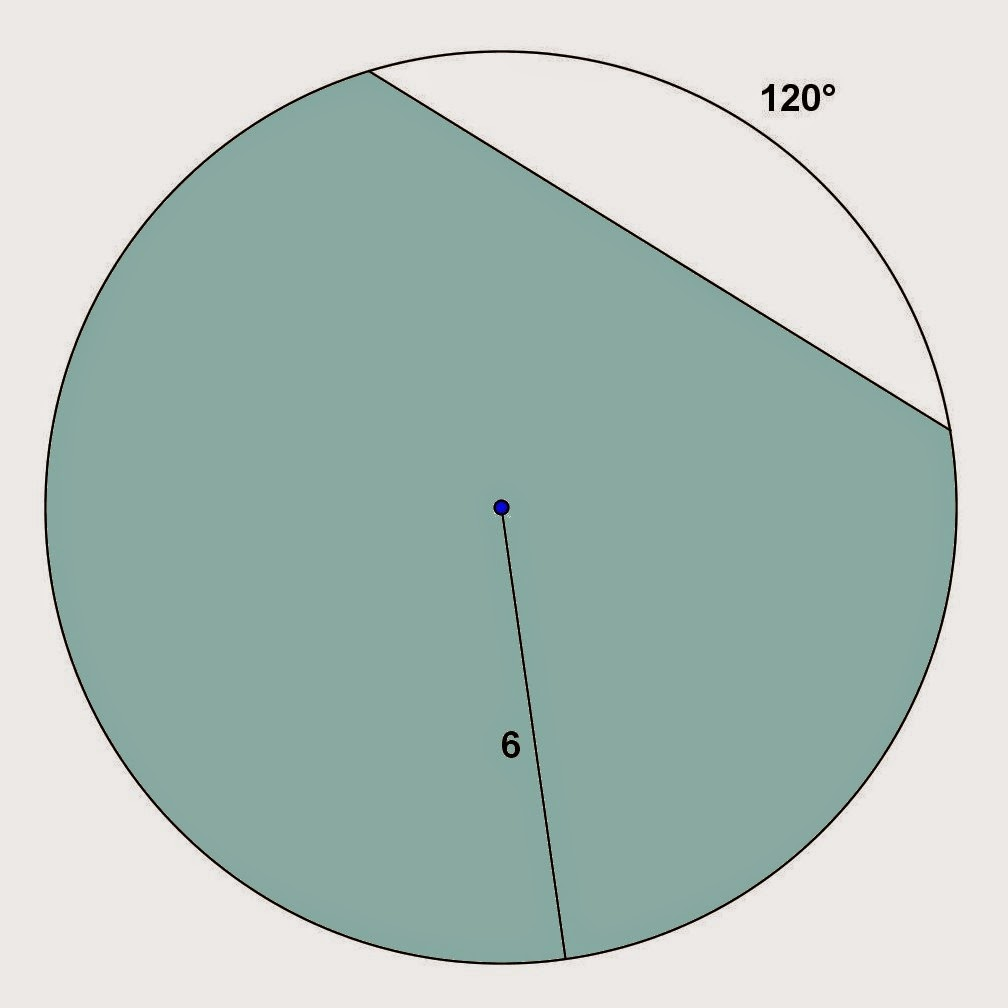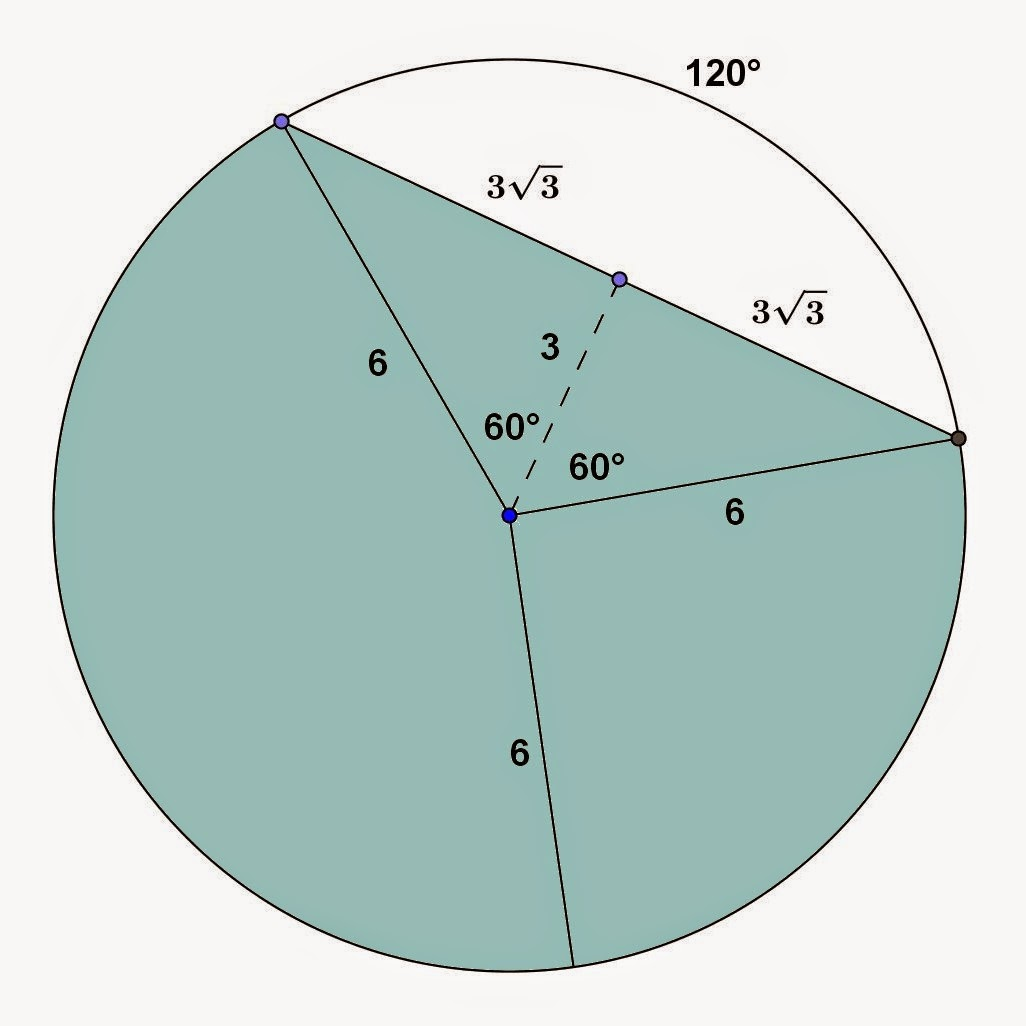## Monday, December 29, 2014

### Circular Segment Problems, 2

Category: Plane Geometry

"Published in Newark, California, USA"

Find the area of the shaded region:Photo by Math Principles in Everyday Life

Solution:

Consider the given figure abovePhoto by Math Principles in Everyday Life

The first thing that we need to do is to label further the given figure so that we can solve for the area of circular segment which is the unshaded portion as followsPhoto by Math Principles in Everyday Life

The angle of an arc is equal to the angle of circular sector which is 120°. If you draw a line which is perpendicular to the given chord, then it becomes two equal right triangles and that line will bisect the angle of circular sector. In this case, there are two 30° - 60° right triangles. Hence, the area of circular segment is

where θ is the angle of circular section in radians (a unit less value of angle). Substitute the values, we have

The area of a circle is

Therefore, the area of shaded region is

or

## Sunday, December 28, 2014

### Square, Rectangle, and Parallelogram Problems, 15

Category: Plane Geometry

"Published in Vacaville, California, USA"

A rectangular parcel of land is 50 ft wide. The length of a diagonal between opposite corners is 10 ft more than the length of the parcel. What is the length of the parcel?

Solution:

To illustrate the problem, it is better to draw the figure as followsPhoto by Math Principles in Everyday Life

If a rectangle is cut by its diagonal, then the resulting figure is a pair of right triangles. By Pythagorean Theorem, we can solve for the value of x as follows

Therefore, the length of a rectangular parcel of land is 120 ft.

## Saturday, December 27, 2014

### Square, Rectangle, and Parallelogram Problems, 14

Category: Plane Geometry

"Published in Vacaville, California, USA"

A rectangular parcel of land has an area of 5000 ft². A diagonal between opposite corners is measured to be 10 ft longer than one side of the parcel. What are the dimensions of the land, correct to the nearest foot?Photo by Math Principles in Everyday Life

Solution:

Consider the given figure abovePhoto by Math Principles in Everyday Life

If a rectangle is cut by its diagonal, then the resulting figure is a pair of right triangles. By Pythagorean Theorem, the width of a rectangle is

Hence, the area of a rectangle is

Square on both sides of the equation, we have

Since the resulting equation cannot be factored by synthetic division, factoring, grouping, or substitution method, then we have to do the trial and error method by assigning the values of x until the left side of the equation equals the right side of the equation which is zero as followsPhoto by Math Principles in Everyday Life

It is better to do the calculations if you will use Microsoft Excel because you can put the formula like for instance, the resulting cubic equation that we can't factor by any method. You can enter any values of x and the Excel program will give us the result as shown at the table above.

From the result as shown at the table above, we have to choose the value of x which is 106.0805885 because the result is closer to zero. Hence, the length of a rectangle is 106.0805885 ft ≈ 106 ft.

The width of a rectangle is

Therefore, the dimensions of the rectangular land are 106 ft by 47 ft